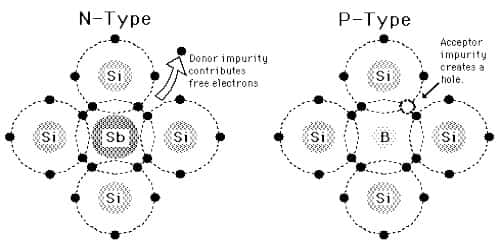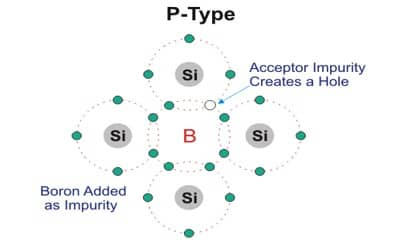Physics

# p-type Semiconductorp-type Semiconductor

If trivalent elements like gallium, aluminum etc. impurities are mixed in intrinsic germanium or silicon in a small amount and in a controlled way then the p-type crystal is formed. Since aluminum has three valence electrons, so these electrons form covalent bonds with three electrons of the adjacent silicon or germanium atom. So, it is seen that the fourth electron of germanium or silicon atom does not form any covalent bond since aluminum has a deficiency of an electron. Due to this deficiency of electron a hole is created in aluminum atom [Figure].Fig: p-type Semiconductor

So, in crystal lattice for each aluminum atom, a hole will be generated and holes generated like this remain eager to accept electrons. For this reason, an aluminum atom is called ‘acceptor’ atom. A number of positively charged holes is manifolding greater than electrons generated due to thermal agitation. Hence in p-type semiconductor positive charges play the main role of current flow, i.e., in this case, holes are majority carriers and electrons are minority carriers. A p-type semiconductor is made by doping an intrinsic semiconductor with Group-III elements such as boron (B) or aluminum (Al). In these elements, there are only three valence electrons per atom. When we use trivalent impurities for doping then we get a p-type semiconductor. Example of trivalent impurities is aluminum or boron.